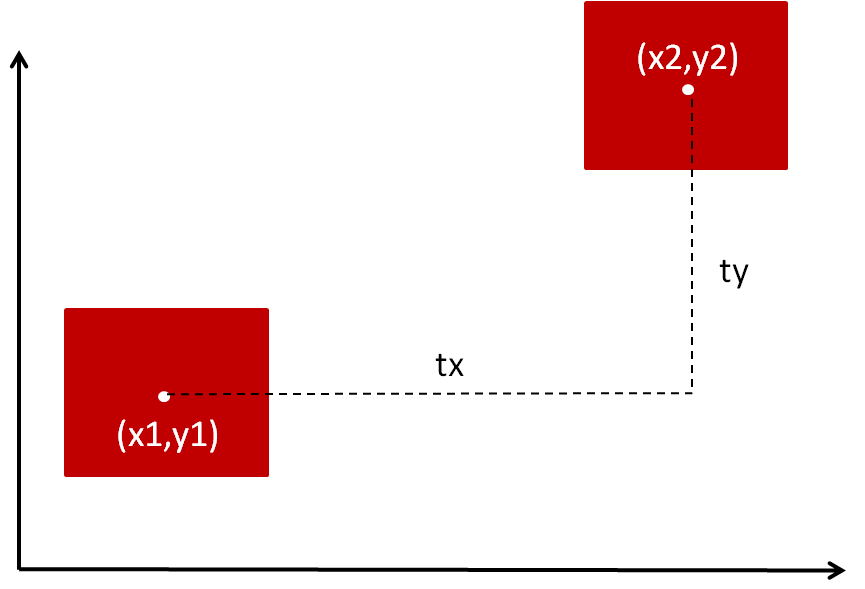# 为什么要引入齐次坐标$x2=x1+tx$
$y2=x2+ty$

$\begin{array}{}\text{(78)}& \left[\begin{array}{c}x2\\ y2\end{array}\right]=\left[\begin{array}{c}x1\\ y1\end{array}\right]+\left[\begin{array}{c}tx\\ ty\end{array}\right]\end{array}$

$\begin{array}{}\text{(79)}& \left[\begin{array}{c}x2\\ y2\end{array}\right]=\left[\begin{array}{cc}{k}_{x}& 0\\ 0& {k}_{y}\end{array}\right]\left[\begin{array}{c}x1\\ y1\end{array}\right]\end{array}$

$\begin{array}{}\text{(370)}& \left[\begin{array}{c}x2\\ y2\end{array}\right]=\left[\begin{array}{cc}cos\theta & -sin\theta \\ sin\theta & cos\theta \end{array}\right]\left[\begin{array}{c}x1\\ y1\end{array}\right]\end{array}$

$\begin{array}{}\text{(371)}& \left[\begin{array}{c}x2\\ y2\end{array}\right]=\left[\begin{array}{cc}0.5& 0\\ 0& 0.5\end{array}\right]\left[\begin{array}{cc}cos45& -sin45\\ sin45& cos45\end{array}\right]\left[\begin{array}{cc}2& 0\\ 0& 2\end{array}\right]\left[\begin{array}{c}x1\\ y1\end{array}\right]\end{array}$

$\begin{array}{}\text{(372)}& \left[\begin{array}{c}x2\\ y2\end{array}\right]=\left[\begin{array}{c}x1\\ y1\end{array}\right]+\left[\begin{array}{c}tx\\ ty\end{array}\right]\end{array}$

$\begin{array}{}\text{(373)}& \left[\begin{array}{c}x2\\ y2\\ 1\end{array}\right]=\left[\begin{array}{ccc}1& 0& tx\\ 0& 1& ty\\ 0& 0& 1\end{array}\right]\left[\begin{array}{c}x1\\ y1\\ 1\end{array}\right]\end{array}$

$\begin{array}{}\text{(374)}& \left[\begin{array}{c}x2\\ y2\\ 1\end{array}\right]=\left[\begin{array}{ccc}{k}_{x}& 0& 0\\ 0& {k}_{y}& 0\\ 0& 0& 1\end{array}\right]\left[\begin{array}{c}x1\\ y1\\ 1\end{array}\right]\end{array}$

$\begin{array}{}\text{(375)}& \left[\begin{array}{c}x2\\ y2\\ 1\end{array}\right]=\left[\begin{array}{ccc}cos\theta & -sin\theta & 0\\ sin\theta & cos\theta & 0\\ 0& 0& 1\end{array}\right]\left[\begin{array}{c}x1\\ y1\\ 1\end{array}\right]\end{array}$

08-093871

#### 为什么要引入齐次坐标，齐次坐标的意义（二）01-071918

#### 为什么要引入齐次坐标？

09-122万+

#### 关于齐次坐标的理解（经典）

07-221万+

#### 为什么要特征标准化及特征标准化方法

03-236万+

#### 程序员为什么千万不要瞎努力？

10-12130

#### 数据库为什么使用B+树而不是B树

12-08682

#### Matlab实验齐次坐标与二维几何变换

02-161074

#### 图形变换和齐次坐标©️2020 CSDN 皮肤主题: 编程工作室 设计师: CSDN官方博客点击重新获取扫码支付1.余额是钱包充值的虚拟货币，按照1:1的比例进行支付金额的抵扣。
2.余额无法直接购买下载，可以购买VIP、C币套餐、付费专栏及课程。余额充值